# Algebra Class 10th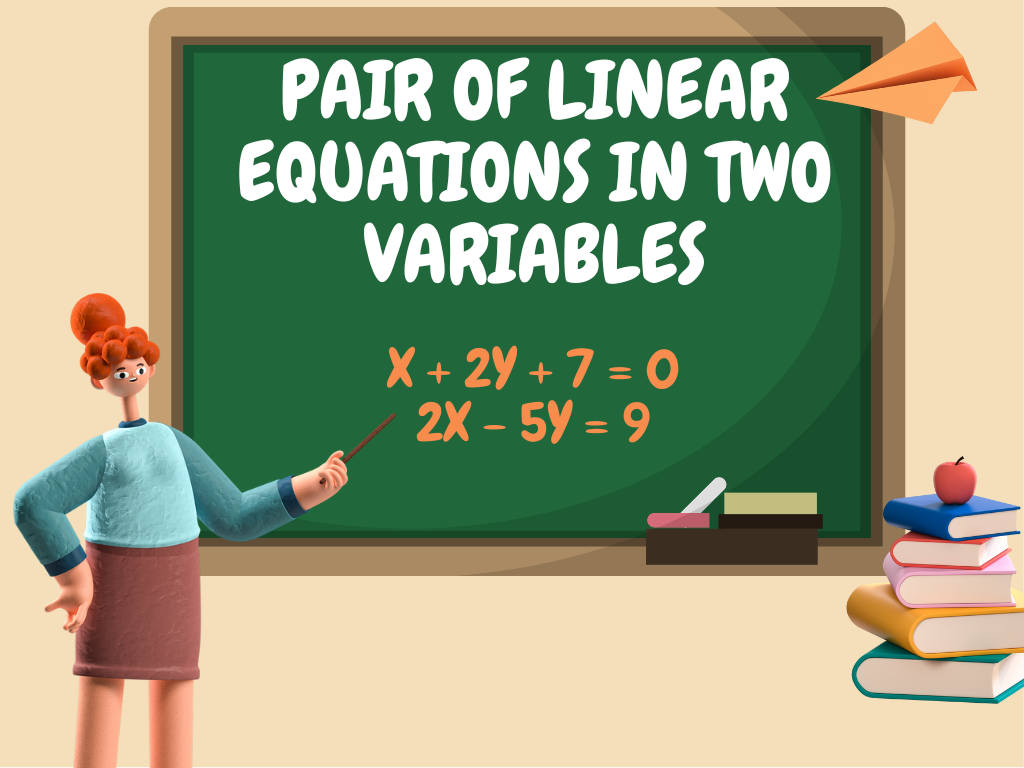## Pair of Linear Equations in Two Variables Class 10th

We are familiar with linear equations in two variables as we have studied them in previous classes. The linear equation having two variables is called the linear equation in two variables. In class 10th, we shall study the pair of linear equations in two variables, the solution of a pair of linear equations in two …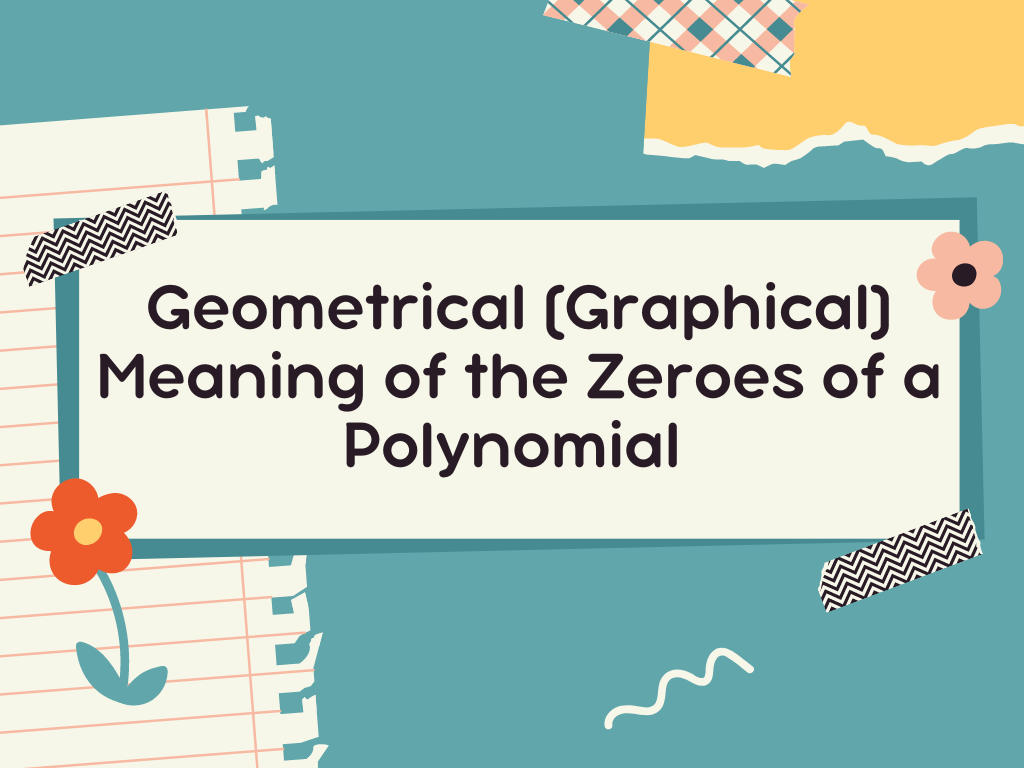## Geometrical (Graphical) Meaning of the Zeroes of a Polynomial Class 10th

Introduction We know that if a polynomial is p(x) and R is it’s zero then p(R) = 0. It means the zeroes of every polynomial satisfy it. Let’s understand this with the help of geometrical or graphical representation. In class 10th, We shall study the geometrical representation of linear polynomials, quadratic polynomials, and cubic polynomials, …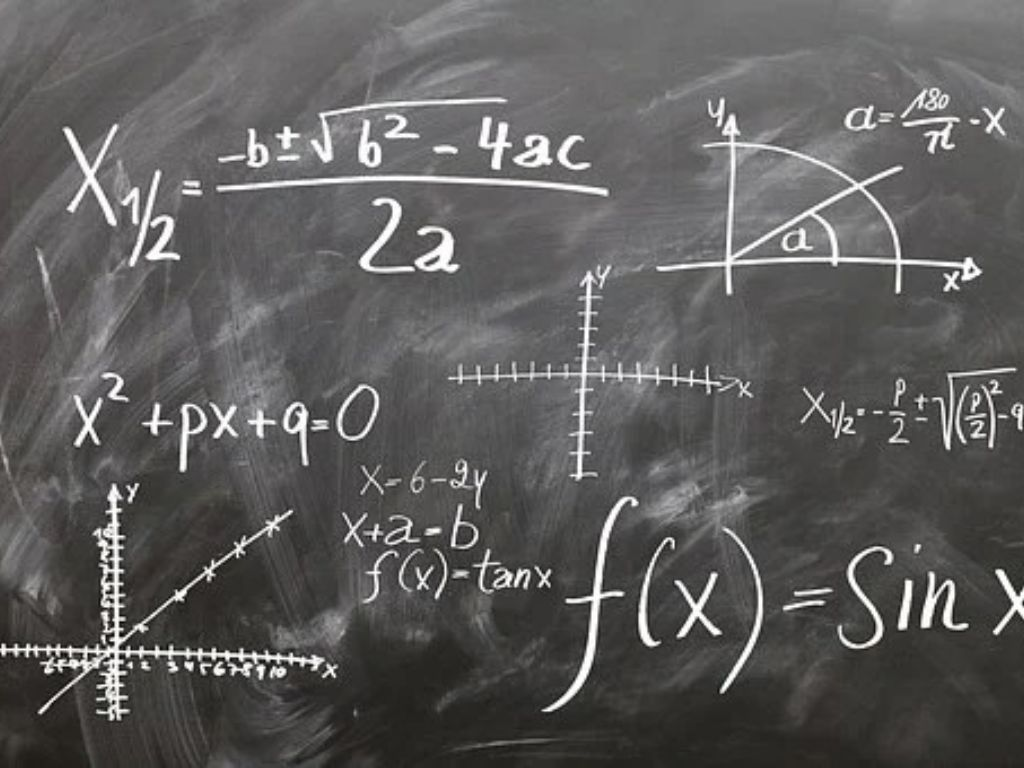## Quadratic Formula (Shridharacharya Sutra) Class 10th

Introduction Quadratic Formula (Shridharacharya Sutra) – The quadratic formula is given by the great Indian mathematician Shridharacharya and is also known as Shridharacharya Sutra. This formula is used to solve the quadratic equation. x = –b ± √(b2 – 4ac)/2a Where, x = variable a, b, c = Coefficients of quadratic equation Derivation of Formula …## Perfect the Square Method Class 10th

Perfect The Square Method (Complete The Square Method) Introduction In the Perfect the Square Method, we convert the quadratic equation into the perfect square form and then we take the square root to obtain the required roots. This method will be clear by the following examples. Examples Example – 1) Solve the quadratic equation 9×2 …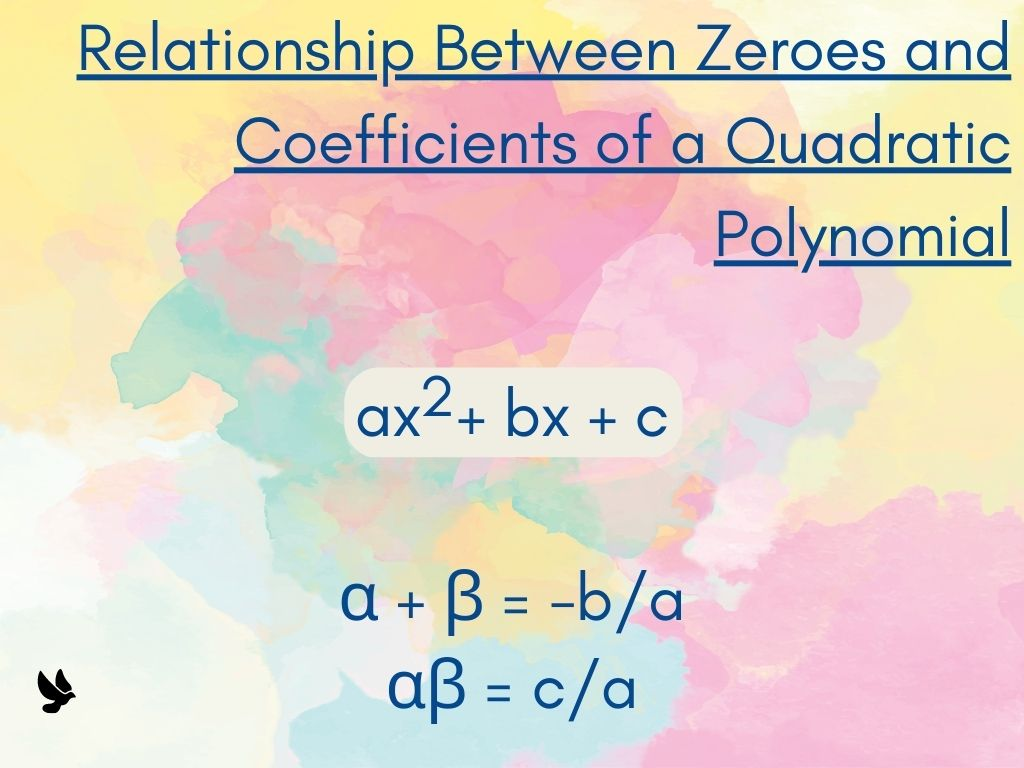## Relationship Between Zeroes and Coefficients of a Quadratic Polynomial Class 10th

Introduction We use the standard form of a quadratic polynomial to find the Relationship between Zeroes and Coefficients of a Quadratic Polynomial. We know the standard form of a quadratic polynomial is f(x) = ax2 + bx + c. Derivation of Formula Let α and β be the two zeroes of this polynomial. Then (x – …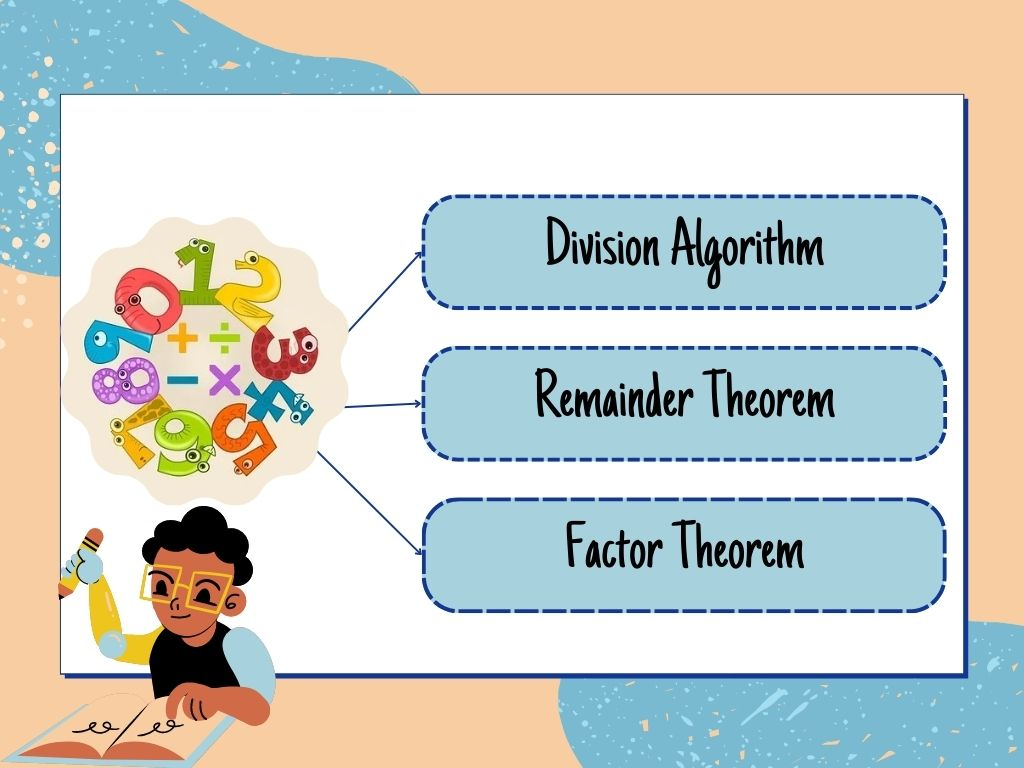## Division Algorithm, Remainder Theorem, and Factor Theorem Class 10th

Introduction Division Algorithm, Remainder Theorem, and Factor Theorem all three are related to Algebra. These three represent the relationship between the two functions. Division Algorithm If p(x) and g(x) are two such polynomials that the degree of p(x) is greater than g(x) and g(x) ≠ 0, and we divide p(x) by g(x) then we get two …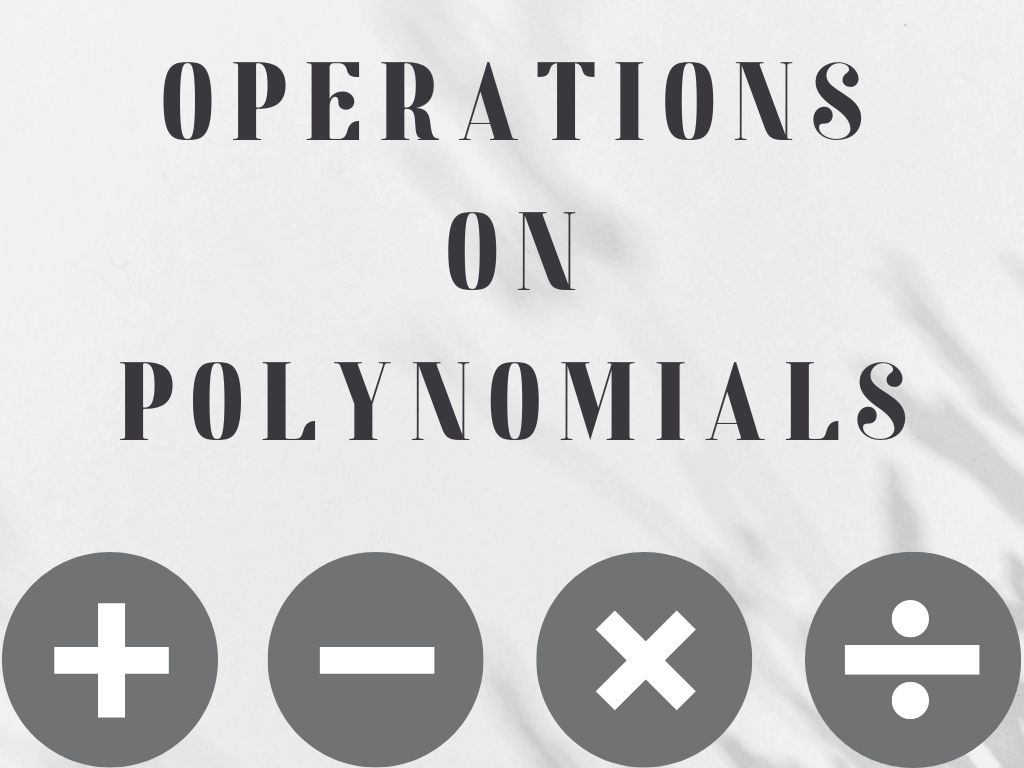## Operations on Polynomials Class 10th

Introduction Operations on polynomials are the addition of polynomials, the subtraction of polynomials, the multiplication of polynomials, and the division of polynomials. ➤ Addition of polynomials ➤ Subtraction of polynomials ➤ Multiplication of polynomials ➤ Division of polynomials Addition of Polynomials When we add two or more polynomials then only like terms are added. it …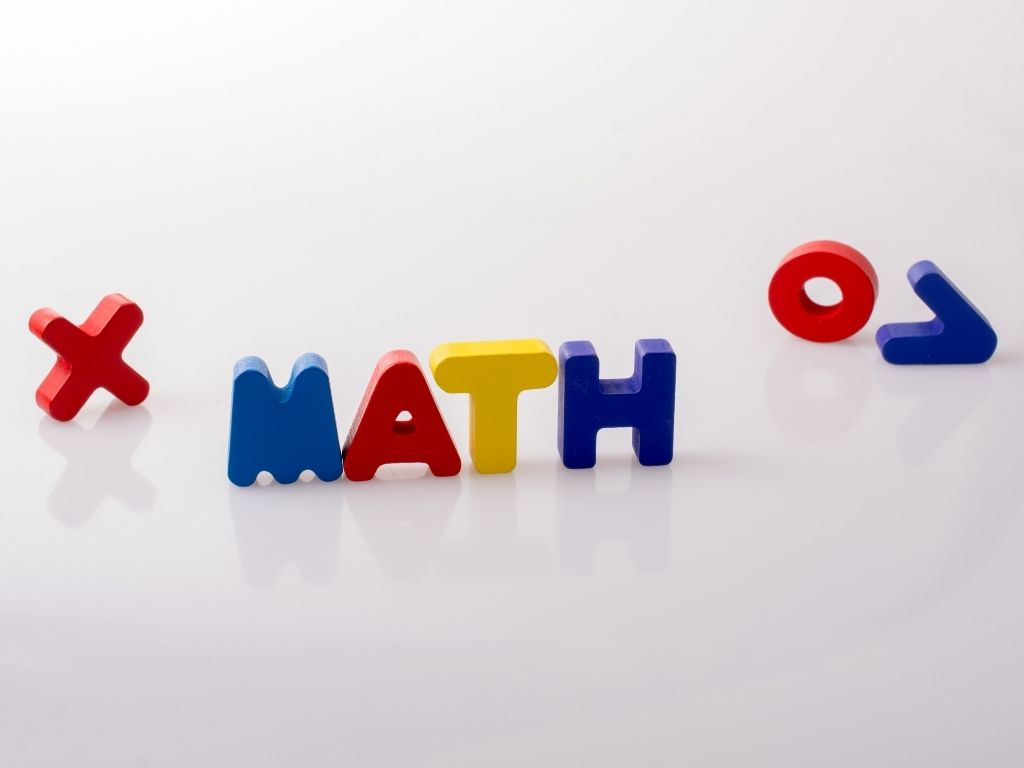## Polynomials Class 10th

Introduction A polynomial word is made up of two Greek terms “poly” which means many and “nominal” which means terms So, a polynomial means many terms. Polynomials are the combined expression of variables, constants, and exponents (only whole numbers) with arithmetic expressions like addition, subtraction, multiplication, and division (division by only constant terms). Polynomials can …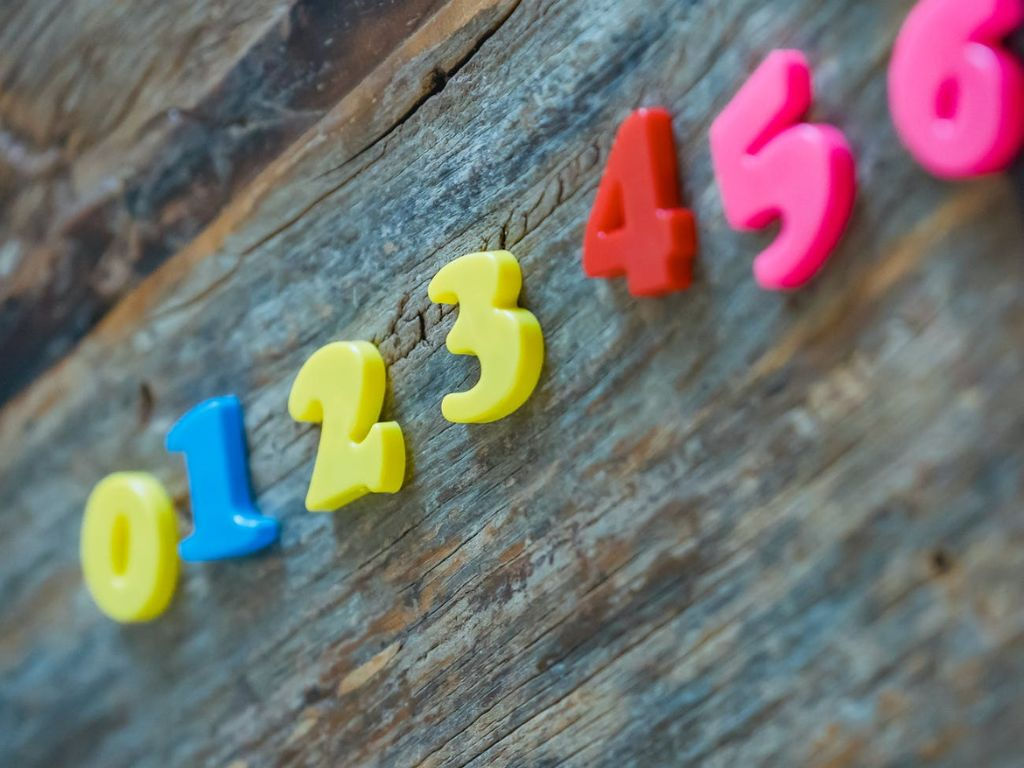## Arithmetic Progression Class 10th

Introduction In our surroundings, we must have observed that many things follow certain patterns or designs such as tiles mounted on the floor at home, stripes of a wheel, Stairs, shapes on watermelon, etc. this certain pattern or design is called a sequence. In some sequence, patterns remain the same but they come repeatedly and …